# How to Convert Parsecs to Light Years

Instructor: Gerald Lemay

Gerald has taught engineering, math and science and has a doctorate in electrical engineering.

In this lesson, we define and relate two very important distance measures: the parsec and the light year. These measures are typically used for describing the immensely long distances between astronomical objects. We'll learn how to convert from parsecs to light years.

## Converting Parsecs to Light Years

Both the parsec (pc) and the light year (LY) are measures of distance useful in astronomy where the distances are huge. For example, the nearest large galaxy cluster is the Virgo Cluster. From the earth, this celestial object is 59 million light years away while the Andromeda Galaxy is about 780 thousand parsecs away.

So let's begin working out the relationship between parsecs and light years!

#### Step 1: Review the definition of the tangent.

For a right-triangle, the tangent of an angle is the opposite side over the adjacent side.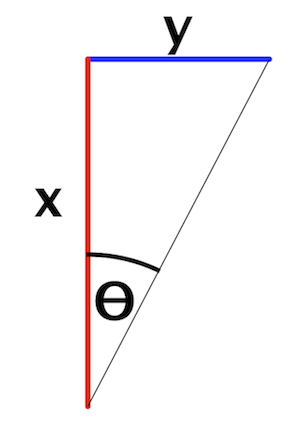In the figure, the tangent of θ is the opposite side, y, divided by the adjacent side, x: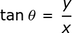#### Step 2: Relate x and y to astronomy.

The astronomical unit (AU) is the average radius of the earth's orbit around the sun. You may know 93 million miles as this number. What if we convert 93 million miles to meters?

• One mile is 1609.3 meters.
• 93 million miles is 93 times 1609.3 million meters.
• This is approximately 1.4960 x 1011 meters.

We use 1.4960 x 1011 meters as the average distance from the sun to the earth. This is 1 AU. In the right-triangle figure, y is 1 AU. The x is the distance we will call 1 parsec (1 pc). Our triangle now looks like: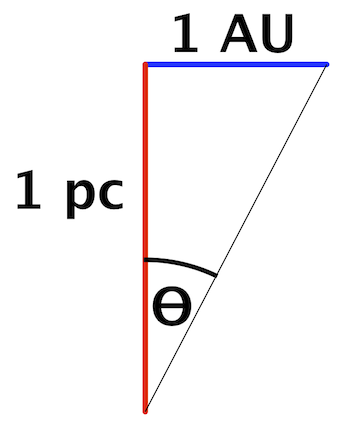In terms of the tangent, just like tan θ is y/x, we now have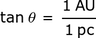#### Step 3: Define the parsec.

The word ''parsec'' is a contraction of ''parallax'' and ''second''. Parallax is the angle subtended at a celestial object by one AU. In our figure, it is the angle θ. And one second of arc is 1/3600 of one degree. This comes from 60 minutes/degree times 60 seconds/minute.

The parsec is the distance when the subtended angle θ is one second of arc.

#### Step 4: Compute tan θ when θ = 1 second of arc.

This is easily computed on a calculator. You can verify the tangent function on your calculator is set to degrees by taking the tangent of 45. The answer should be 1.#### Step 5: Calculate the number of meters in 1 pc.

Remember tan θ = 1 AU/1 pc? Then, 1 pc = 1 AU/tan θ (when θ = 1/3600 degrees). In the calculation, we also use 1.4960 x 1011 meters as the value of AU.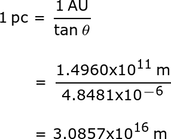#### Step 6: Calculate how many seconds of time in 1 year.

This is a fun conversion exercise. We use:

• 365 days
• 24 hours/1 day
• 60 minutes/1 hour
• 60 seconds/1 minute

To unlock this lesson you must be a Study.com Member.

### Register to view this lesson

Are you a student or a teacher?